Courses

# Test: Mental Ability, Analytical & Logical Reasoning - 6 (2015-2015)

## 20 Questions MCQ Test UPSC Topic Wise Previous Year Questions | Test: Mental Ability, Analytical & Logical Reasoning - 6 (2015-2015)

Description
This mock test of Test: Mental Ability, Analytical & Logical Reasoning - 6 (2015-2015) for UPSC helps you for every UPSC entrance exam. This contains 20 Multiple Choice Questions for UPSC Test: Mental Ability, Analytical & Logical Reasoning - 6 (2015-2015) (mcq) to study with solutions a complete question bank. The solved questions answers in this Test: Mental Ability, Analytical & Logical Reasoning - 6 (2015-2015) quiz give you a good mix of easy questions and tough questions. UPSC students definitely take this Test: Mental Ability, Analytical & Logical Reasoning - 6 (2015-2015) exercise for a better result in the exam. You can find other Test: Mental Ability, Analytical & Logical Reasoning - 6 (2015-2015) extra questions, long questions & short questions for UPSC on EduRev as well by searching above.
QUESTION: 1

### Geeta: Naresh has become a better boxer since he started meditation. Radha: Impossible, A boxer's most important asset is his aggressiveness. Radha's statement reflects her belief that 

Solution:

Radha did not accept the argument that a person becomes a better boxer after meditation. The reason for this is that a boxer, according to her, should be aggressive. This indicates that Radha believes that meditation makes a person less aggressive.

QUESTION: 2

### Consider the following statements : 1. A man had a wife, two sons and daughters in his family 2. The daughters were invited to a feast and the male members of the family went out to take part in a picnic 3. The man's father did not return from his work. Which of the following statements is true? 

Solution: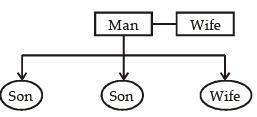All the male member of the family went out to take part in a picnic. It is given that daughters were invited to a feast.
Nothing is specified about men’s wife. Hence, the statement that the man’s wife is likely to be left at home is true.

QUESTION: 3

### Four persons A, B, C and D consisting of two married couples are in a group. Both the women are shorter than their respective husbands. A is the tallest among the four. C is taller than B. D is B's brother. In this context, which one of the following statements is not correct? 

Solution:

Given, A is tallest among the four. Hence, A is male, D is also male, as D is the brother of B. Then, B and C are females. D is the husband of C. D is the shorter than A and is taller than B. These, B is the shorter from above only (c) is incorrect.

QUESTION: 4

A father is nine times as old as his son and the mother is eight times as old as the son. The sum of the father's and the mother's age is 51 years. What is the age of the son?



Solution:

Let age of son = x years
Age of father = 9x
Age of mother = 8x
Now, According to question,
8x + 9x = 51
17x = 51
x = 3 years

QUESTION: 5

In a test, a candidate attempted only 8 questions and secured 50% marks in each of the questions. If he obtained a total of 40% in the test and all questions in the test carried equal marks, how many questions were there in the test?



Solution:

Let marks of each question = x
According to question,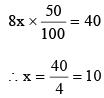∴ Total questions in test = 100/10 = 10

QUESTION: 6

Four cardboard pieces of specific shapes are shown in the following figure: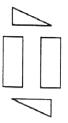Which one of the following figures given can be formed by joining these pieces together?



Solution:

By combining the given fragment, we get figure (d).

QUESTION: 7

What is the missing number 'X' of the series 7, X, 21, 31, 43?



Solution: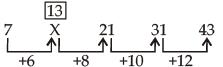QUESTION: 8

Consider the following matrix: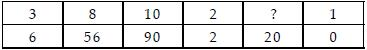What is the missing number at '?' in the matrix?



Solution:

In each column, let the number at the top be ‘x’ and the one at the bottom is ‘(x – 1)’. The relation between columns are given by
(x) (x – 1) = 3(3 – 1) = 6
Therefore, 5(5 – 1) = 20

QUESTION: 9

Consider the figures given below: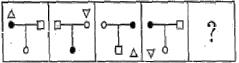To fit the question mark, the correct answer is



Solution:

The ‘Δ’ is shifting one corner to the next in the clockwise direction and each time it is getting inverted.
Thus, the Δ should appear at the top left corner pointing upward. Rest of the elements are shifting positions in the counter clockwise direction.

QUESTION: 10

"By liberty I mean the eager maintenance of that atmosphere in which men have the opportunity to be their best selves."
Which one of the following expresses the view implied in the above statement?



Solution:

(a) is wrong because the sentence says that liberty means the eager maintenance of that atmosphere which might require restrictions as well.
(b) is wrong because if the people work only according to the law they might become good citizens but might not work to their best selves.
(c) is wrong because if people do what they desire it might lead to materialist thinking which is not the best self.
(d) is correct because with the growth of human personality the people would be their best selves.

QUESTION: 11

The mangroves can shed tons of leaves per acre every year; fungi and bacteria break down this leaf litter and consume it, they then are consumed by tiny worms and crustaceans, which in turn feed small fish, which feed larger fish and birds and crocodiles.
Which among the following is the most logical inference of the above statement?



Solution:

The passage clearly talks about the role Mangroves play in some of the coastal food chains. Other food chains - with or without Mangroves - might exist but they are not mentioned in the passage. So (c) is correct. The passage does not say that no food chain is possible without Mangroves so (a) is wrong. (b) is also wrong as the passage does not talk about Mangroves being an essential component of all marine ecosystems. Further (d) is also wrong for the same reasoning as the passage does not talk about the composition of marine flora and fauna.

QUESTION: 12

In a parking area, the total number of wheels of all the cars (four-wheelers) and scooters/motorbikes (two-wheelers) is 100 more than twice the number of parked vehicles. The number of cars parked is



Solution:

Let number of cars be x
Let number of scooters / motor bikes = y
∴ Total number of parked vehicles = x + y
According to question
4x + 2y = 2 (x + y) + 100
4x + 2y = 2 x + 2y + 100
2x = 100
x = 50
Hence, Number of cars parked is 50.

QUESTION: 13

In a group of persons travelling in a bus, 6 persons can speak Tamil, 15 can speak Hindi and 6 can speak Gujarati. In that group none can speak any other language. If 2 persons in the group can speak two languages only and one person can speak all the three languages, then how many persons are there in the group?



Solution:

Let a = Speaks one language
Let b = Speaks two language
Let c = Speaks three language then
a + 2b + 3c = 6 + 15 + 6
a + 4 + 3 = 27
a = 20
∴ Total number of persons in the group
= 20 + 2 + 1 = 23.

QUESTION: 14

Out of 130 students appearing in an examination, 62 failed in English, 52 failed in Mathematics, whereas 24 failed in both English and Mathematics. The number of students who passed finally is



Solution: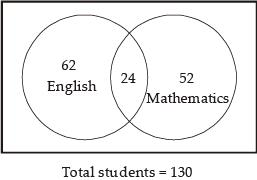Total students failed = (62 + 52) – 24
= 114 – 24
= 90
Number of students passed  finally = 130 – 90 = 40

QUESTION: 15

Twelve people form a club. By picking lots, one of them will host a dinner for all once in a month. The number of dinners a particular member has to host in one year is



Solution:

Cannot be predicted because data is not sufficient.

QUESTION: 16

Shahid and Rohit start from the same point in opposite directions. After each 1 km, Shahid always turns left and Rohit always turns right. Which of the following statements is correct?



Solution:

After both have travelled 3 km, their positions would be on follows.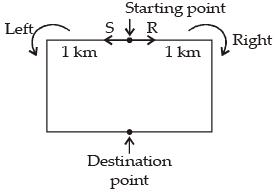Hence, (b) is correct.

QUESTION: 17

Examine the following statements:
1. Lady's finger is tastier than cabbage.
2. Cauliflower is tastier than lady's finger.
3. Cabbage is not tastier than peas.
The conclusion that can be drawn from these statements is that



Solution:

Cauliflower > Lady finger > Cabbage
But Peas > Cabbage
The conclusion that can be drawn from these statements is that cabbage is the least tasty among the four vegetables.

QUESTION: 18

Each of A,B, C and D has Rs. 100. A pays Rs. 20 to B, who pays Rs. 10 to C, who gets Rs. 30 from D. In this context, which one of the following statements is not correct?



Solution:

According to question,
Each of A, B, C and D = 100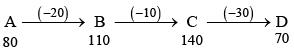So, option (c) is correct.

QUESTION: 19

If A runs less fast than B, and B runs as fast but not faster than C; then, as compared to A, C runs



Solution:

A < B < C
Now, compared to A, C runs faster than A.

QUESTION: 20

A society consists of only two types of people fighters and cowards. Two cowards are always friends. A fighter and a coward are always enemies. Fighters are indifferent to one another. If A and B are enemies, C and D are friends, E and F are indifferent to each other, A and E are not enemies, while B and F are enemies.
Which of the following statements is correct?



Solution: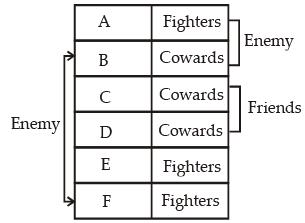From above table, it is clear that A, E and F are fighters.